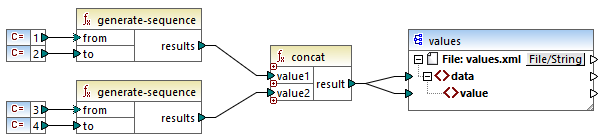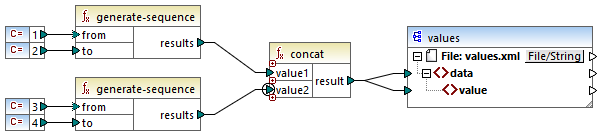# Altova MapForce 2020 Professional Edition

 Priority context Scroll Home Prev Top Next More

Priority context is a way to influence the order in which input parameters of a function are evaluated. Setting a priority context may be necessary if your mapping joins data from two unrelated sources.

To understand how priority context works, recall that, when a mapping runs, the connection to an input item may carry a sequence of multiple values. For functions with two input parameters, this means that MapForce must create two loops, one of which must be processed first. The loop that is processed first is the "outer" loop. For example, the equal function receives two parameters: a and b. If both a and b get a sequence of values, then MapForce processes as follows:

For each occurrence of a

oFor each occurrence of b

Is a equal to b?

As you can see from above, each b is evaluated in the context of each a. Priority context lets you alter the processing logic so that each a is evaluated in the context of each b. In other words, it lets you swap the inner loop with the outer loop, for example:

For each occurrence of b

oFor each occurrence of a

Is a equal to b?

Let's now examine a mapping where priority context affects the mapping output. In the mapping below, the concat function has two input parameters. Each input parameter is a sequence that was generated with the help of the generate-sequence function. The first sequence is "1,2" and the second sequence is "3,4".First, let's run the mapping without setting a priority context. The concat function starts evaluating the top sequence first, so it combines values in the following order:

1 with 3

1 with 4

2 with 3

2 with 4

This is reflected in the mapping output as well:

   13   14   23   24

If you right-click the second input parameter and select Priority Context from the context menu, it will become the priority context. As illustrated below, the priority context input is encircled.This time, the second input parameter will be evaluated first. The `concat` function will still concatenate the same values, but this time it will process the sequence `3,4` first. Consequently, the output becomes:

   13   23   14   24

So far, you have seen only the theoretical part behind priority context. For a more practical scenario, see Example: Filter with priority context.